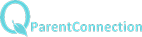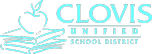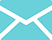Statistics and ProbabilityChapter 13

Outline

Java Applets

Chapter 13

Main Topics

13.1 Test for Goodness of Fit

A. Chi-Squared Distribution

1. The Chi-Squared Distribution is skewed right.

2. Must use Degree’s of Freedom

3. As the sample size increases, the curve spreads

out

B. The test is to verify whether or not a distribution of a

population is the same as the hypothesized amounts.

1. The Null Hypothesis

a. The actual proportions is equal to the

hypothesized proportions

2. Alternative Hypothesis

a. The actual proportions is different from the

hypothesized proportions

3. Conditions

a. All expected counts are at least 1 or above

b. No more than 20% of the expected counts are

less than five

13.2 Inference for Two-Way Tables

A. Two-Way Tables

1. Dimensions are r x c (row and column)

2. Expected counts are calculated by product of row

total and column total divided by the entire total.

3. Use the same equation as Test for Goodness of Fit.

4. Degree of Freedom is calculated by (r-1)(c-1).

B. Test for Homogeneity of Populations

1. Test is reveal if the proportions for each group are

the same.

C. Test for Independence.

1. Same test as Homogeneity, but the result is to

determine if one category influences another

category.

AP Statistics

Syllabus

Buchanan High School     |     1560 N. Minnewawa Ave.    |     Clovis, CA     |     93619     |     (559) 327-3000